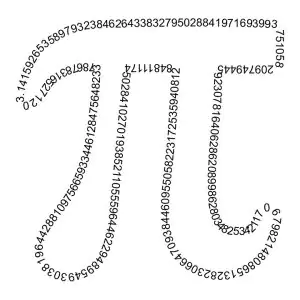Pi is usually known as a string of numbers starting from 3.14. However, it is much more than that. It is a mathematical constant. Originally, it was defined as the ratio of a circle’s circumference to its diameter. However, it now has various definitions and is in many formulas in mathematics and physics. Though it is called “pi” in English, it commonly represented by the Greek letter “π.” Anyway, in the following paragraphs, we will go into detail about its fundamentals, history, and role.One-stop solution for all your homework needs. Get the job done.

✅ AI Essay Writer ✅ AI Detector ✅ Plagiarism checker ✅ Paraphraser

Pi is the most known mathematical constant. As was mentioned in the introduction, it equals the circumference of a circle divided by the diameter. According to LiveScience, “No matter how large or small a circle is, pi will always work out to be the same number. That number equals approximately 3.14, but it’s a little more complicated than that” (Hom, Elaine J.). Pi is also noted as an irrational number, which basically points to the fact that is a real number that can be shown as a simple fraction. In fact, it is what mathematicians call an “infinite decimal,” as pi’s digits go on forever. Also, there are no repeating patterns in pi.

Since pi’s digits go on forever, mathematicians and scientists often approximate it. According to Wonderopolis, “For most purposes, pi can be approximated as 3.14159. Some people even shorten it to 3.14, which is why Pi Day is celebrated on March 14 (3/14)” (“What Is Pi?”). What is interesting is that computers have calculated pi to over three trillion digits. Some people even remember 1000s of digits of pi as a mental exercise.

But where did this number come from? Surprisingly, this formula has been in use for over 4000 years. The first people to discover pi was the Babylonians. According to Exploratorium, “The ancient Babylonians calculated the area of a circle by taking 3 times the square of its radius, which gave a value of pi = 3. One Babylonian tablet (ca. 1900–1680 BC) indicates a value of 3.125 for π, which is a closer approximation. The Egyptians calculated the area of a circle by a formula that gave the approximate value of 3.1605 for π” (“A Brief History of Pi (π)”). However, the first calculation of pi was completed by Archimedes (287–212 BC). Yet, he knew he did not find the value of pi, but rather an approximation of its value, with him stating that pi was between 3 1/7 and 3 10/71.

Pi has many roles. In mathematics, “Most geometry students first encounter pi when they study circles and learn that the area of a circle is equal to pi times the square of the length of the radius.” It is also used widely in trigonometry. Formulae concerning circles, spheres, or ellipses commonly use pi. Here are some common formulae that use it:
The circumference of a circle with radius r is 2πr.
The area of a circle with radius r is πr2.
The volume of a sphere with radius r is 4/3πr3.
The surface area of a sphere with radius r is 4πr2.
(A Guide Book to Mathematics)

In physics, pi is also commonplace. According to LiveScience, “Pi also appears in the physics that describes waves, such as ripples of light and sound. It even enters into the equation that defines how precisely we can know the state of the universe, known as Heisenberg’s uncertainty principle. A river’s windiness is determined by its “meandering ratio,” or the ratio of the river’s actual length to the distance from its source to its mouth as the crow flies” (Wolchover, Natalie). Therefore, pi has many applications in physics as well.

Pi is surprisingly in popular culture. There is an even a day celebrated for this mathematical constant. However, most do not know that it was first discovered by the ancient Babylonians and refined by Archimedes. Also, many people do not know that it is an irrational number that equals the circumference of a circle divided by the diameter. This special formula has many applications in mathematics, physics, and even more areas.

## Works Cited

Hom, Elaine J. “What Is Pi?” LiveScience, Purch, 19 Oct. 2018, www.livescience.com/29197-what-is-pi.html.

“What Is Pi?” Wonderopolis, wonderopolis.org/wonder/what-is-pi.

“A Brief History of Pi (π).” Exploratorium, 5 Mar. 2019, www.exploratorium.edu/pi/history-of-pi.

A Guide Book to Mathematics. Springer Verlag, 2012.

Wolchover, Natalie. “What Makes Pi So Special?” LiveScience, Purch, 9 Aug. 2012, www.livescience.com/34132-what-makes-pi-special.html.